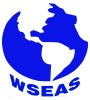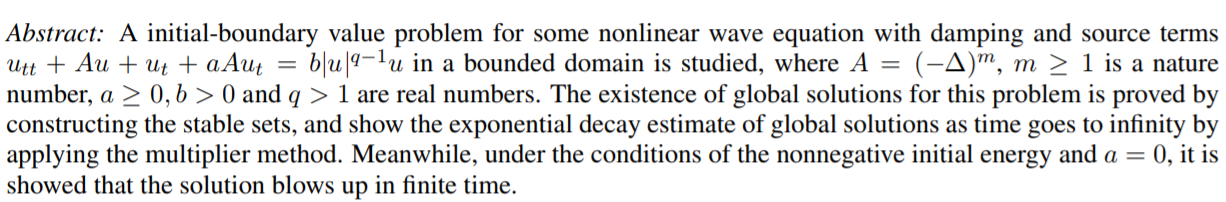Yaojun Ye

### Authors and WSEAS

Yaojun Ye# WSEAS Transactions on Mathematics

Print ISSN: 1109-2769
E-ISSN: 2224-2880

Volume 17, 2018

Notice: As of 2014 and for the forthcoming years, the publication frequency/periodicity of WSEAS Journals is adapted to the 'continuously updated' model. What this means is that instead of being separated into issues, new papers will be added on a continuous basis, allowing a more regular flow and shorter publication times. The papers will appear in reverse order, therefore the most recent one will be on top.

Volume 17, 2018

Global Existence and Exponential Decay of Solutions for a Class of Nonlinear Wave Equations

AUTHORS: Yaojun YeKEYWORDS: Nonlinear wave equation; Initial boundary value problem; Stable sets; Nonlinear damping and source terms; Exponential decay

REFERENCES:

 A.Benaissa and S.A.Messaoudi,Exponential decay of solutions of a nonlinearly damped wave equation, Nonlinear Differ. Equ. Appl., 12(2005), 391-399.

 P. Bernner and W. Von Whal, Global classical solutions of nonlinear wave equations, Math. Z., 176 1981, 87-121.

 A.B.Bijan and B.H.Lichaei, Existence and nonexistence of global solutions of the Cauchy problem for higher order semilinear pseudohyperbolic equations, Nonlinear Analysis TMA, 72(2010), 3275-3288.

 W.Y.Chen and Y.Zhou, Global nonexistence for a semilinear Petrovsky equation, Nonlinear Anal., 70(2009), 3203-3208.

 V.Georgiev and G.Todorova, Existence of solutions of the wave equation with nonlinear damping and source terms, J. Diff. Eqns., 109(1994), 295-308.

 V. Komornik, Exact Controllability and Stabilization, The Multiplier Method, RAM: Research in Applied Mathematics, Masson-John, Wiley: Paris, 1994.

 Y.C.Liu and J.S.Zhao, On potential wells and applications to semilinear hyperbolic equaWSEAS tions and parabolic equations, Nonlinear Anal. ,64(2006), 2665-2687.

 X.F.Liu and Y.Zhou, Global nonexistence of solutions to a semilinear wave equation in the Minkowski space, Appl. Math. Lett., 21 (2008), 849-854.

 S.A.Messaoudi, On the decay of solutions for a class of quasilinear hyperbolic equations with nonlinear damping and source terms, Math. Methods Appl. Sci., 28(2005), 1819-1828.

 S.A.Messaoudi, Global existence and nonexistence in a system of Petrovsky, J. Math. Anal. Appl., 265(2002), 296-308.

 C.X.Miao, The time space estimates and scattering at low energy for nonlinear higher order wave equations, ACTA Mathematica Sinica, Series A, 38 (1995), 708-717.

 M. Nakao, Bounded, periodic and almost periodic classical solutions of some nonlinear wave equations with a dissipative term, J. Math. Soc. Japan, 30(1978), 375-394.

 M. Nakao and H. Kuwahara, Decay estimates for some semilinear wave equations with degenerate dissipative terms, Funkcialaj Ekvacioj, 30(1987), 135-145.

 H. Pecher, Die existenz regulaer L ¨ osungen f ¨ ur¨ Cauchy-und anfangs-randwertproble-me michtlinear wellengleichungen, Math. Z., 140(1974), 263-279.

 L.E.Payne and D.H.Sattinger, Saddle points and instability of nonlinear hyperbolic equations, Israel J. Math., 22(1975), 273-303.

 D.H. Sattinger, On global solutions for nonlinear hyperbolic equations, Arch. Rational Mech. Anal., 30(1968), 148-172.

 E.Vitiliaro, Global nonexistence theorems for a class of evolution equations with dissipation, Arch. Rational Mech. Anal., 149(1999), 155- 182.

 B.X.Wang, Nonlinear scattering theory for a class of wave equations in Hs , J. Math. Anal. Appl., 296 (2004), 74-96.

 Yang Z, Existence and asymptotic behavior of solutions for a class of quasilinear evolution equations with nonlinear damping and source terms, Math. Methods Appl. Sci., 25(2002), 795- 814.

 Y.J.Ye, Existence and asymptotic behavior of global solutions for a class of nonlinear higherorder wave equation, Journal of Inequalities and Applications, 2010(2010), 1-14.

 Y.Zhou, Global existence and nonexistence for a nonlinear wave equations with damping and source term, Math. Nachr., 278(2005), 1341- 1358.

 Y.Zhou, Global nonexistence for a quasilinear evolution equation with critical lower energy, Arch. Inequal. Appl., 2 (2004), 41-47.

 Y.Zhou, Global nonexistence for a quasilinear evolution equation with a generalized Lewis function, Z. Anal. Anwendungen, 24 (2005), 179-187.

WSEAS Transactions on Mathematics, ISSN / E-ISSN: 1109-2769 / 2224-2880, Volume 17, 2018, Art. #31, pp. 252-257